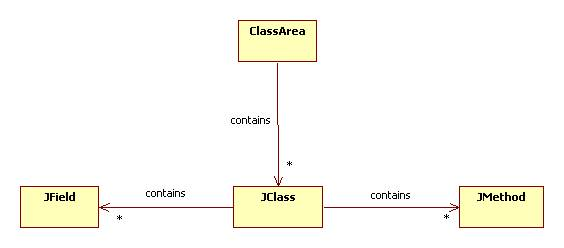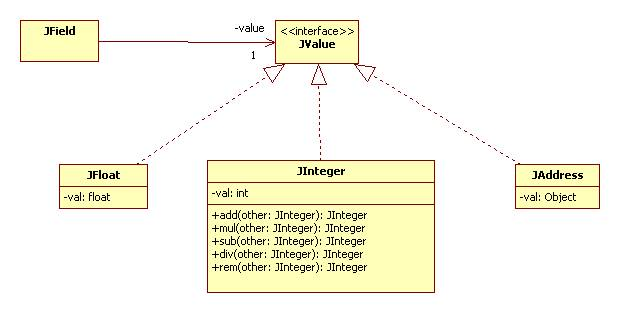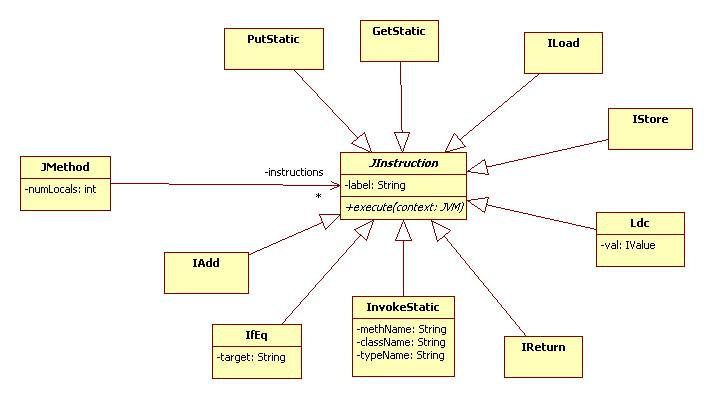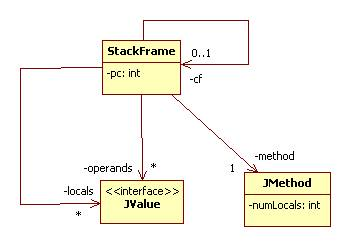# A Java Implementation of the Java Virtual Machine (JVM)

## JVM Organization

This JVM simplifies the actual JVM by not supporting native method invocations (NMI) and multi-threading.

We can view the JVM as a virtual processor with access to three memory areas:Classes, methods, and static fields reside in the class area.

Objects reside in the heap.

Parameters and local variables reside in the stack frame, which can be viewed as the top frame in a "stack" of frames linked by pointers ("cf" in the diagram). The stack is referred to as the Java stack.

Here's a sketch of the JVM:

public class JVM {

private StackFrame topFrame;
private Heap heap;
private ClassArea classes;

public void run() {
while(topFrame != null) {
JInstruction next = topFrame.nextInstruction();
next.execute(this);
}
}
}

## The Class Area

The class area contains a map that associates names of classes to instances of JClass:

public class ClassArea {
private Map<String, JClass> classes;
// etc.
}

A JClass instance contains two maps, one that associates names to static fields, and another that associates names to methods (both static and virtual):

public class JClass {
private Map<String, JField> fields;
private Map<String, JMethod> methods;
// etc.
}

We can approximate this arrangement with the following UML diagram:## Fields and Data Types

A (static) field contains a value. All JVM values have type JValue:In the diagram above we show three concrete subtypes of JValue: JFloat, JAddress, and JInteger. Each is a wrapper for a corresponding Java value. Of course other JValues corresponding to longs, doubles, chars, etc. are possible.

## Methods and Instructions

A method (JMethod instance) has a numLocals attribute that tells us how many parameters and locals it uses. It also has a list of instructions:We equate our JVM instruction set to the Jasmin instruction set.

Note that each instruction has a label (= null if no label) and knows how to execute itself when supplied with a JVM.

Here's a sketch of JInstruction:

public abstract class JInstruction {
private String label; // = null if no label
public abstract void execute(JVM context);
// etc.
}

For an example of a concrete subclass, here's a declaration of IAdd:

public class IAdd extends JInstruction {
public IAdd(String label) { super(label); }

public void execute(JVM context) {
// get operands stack from the JVM's top frame:
Stack<JValue> stack = context.getTopFrame().getOperands();

if (stack.size() < 2) {
System.out.println("not enough operands on stack");
System.exit(1);
}

// remove top 2 items from stack
JValue val1 = stack.pop();
JValue val2 = stack.pop();

if (!(val1 instanceof JInteger) ||
!(val2 instanceof JInteger)) {
System.exit(1);
}

JInteger int1 = (JInteger)val1;
JInteger int2 = (JInteger)val2;

// push sum onto stack:
stack.push(result);
}
}

## Stack Frames

A stack frame is created and becomes a JVM's new topFrame each time the invokestatic instruction is executed.

A stack frame has a pointer to the method being executed (method) as well as a field called pc, which equals the index of the next instruction in the frame's method that is to be executed.

A stack frame has a pointer (cf) to the previous top frame. This stack frame once again becomes the top frame when ireturn or return is executed.

A stack frame has an array for storage of parameters and local variables (locals). The length of this array is given by the numLocals attribute of the frame's method.

Finally, the operand stack (operands) is used to store intermediate values during a computation. For example, the iadd instruction replaces the top two elements of the operand stack by their sum.Here's a sketch of the StackFrame declaration:

public class StackFrame {
private Stack<JValue> operands;
private JValue[] locals;
private int pc;
private JMethod method;
private StackFrame cf;
// etc.
}

## The Assignment

Complete the implementation of the JVM. To simplify matters, you may assume no objects. This means the heap is a null class:

class Heap { }

It also means all methods and fields are static. Further, you may assume the only type is JInteger (i.e., int).

Here are the Jasmin instructions your JVM needs to be able to execute:

Data Control:
getstatic
putstatic
istore
ldc

Sequence Control:
ifeq
ifgt
goto
invokestatic
ireturn
return

Arithmetic: